# AP Calculus AB : An intuitive understanding of continuity

## Example Questions

### Example Question #1 : An Intuitive Understanding Of Continuity

Is the following piecewise function continuous for all? If not, state where it is discontinuous.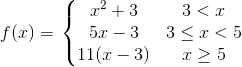No. The function is not continuous at bothand.

No. The function is not continuous at.

Yes. The function is continuous at all.

No. There are sharp turns atand.

No. The function is not continuous at.

Yes. The function is continuous at all.

Explanation:

To check if the piecewise function is continuous, all we need to do is check that the values at 3 and 5 line up.

At, this means checking that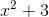and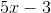have the same value. More formally, we are checking to see that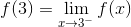, as to be continuous at a point, a function's left and right limits must both equal the function value at that point.

Plugging 3 into both, we see that both of them are 12 at. Thus, they meet up smoothly.

Next, for, we haveand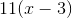. Plugging in 5, we get 22 for both equations.

As all three equations are polynomials, we know they will be continuous everywhere else, and they meet up smoothly at the piecewise bounds, thus ensuring that the function is continuous everywhere.

Note, there are sharp turns atand, but this only means the function isn't differentiable at these points -- we're only concerned with continuity, which is if the equations meet up. Thus, the function is continuous.

### Example Question #1 : An Intuitive Understanding Of Continuity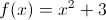when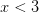and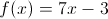when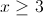At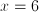the funciton described above is:

undefined

neither differentiable or continuous

differentiable but not continuous

both continuous and diffentiable

continuous but not differentiable

both continuous and diffentiable

Explanation:

If graphed the student will see that the two graphs are continuous at. There is no gap in the graph or no uneven transitions. If the graph is continuous then it is differentiable so it must be both.

### Example Question #3 : An Intuitive Understanding Of Continuity

Which of the following functions contains a removeable discontinuity?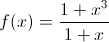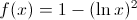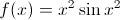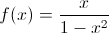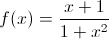Explanation:

A removeable discontinuity occurs whenever there is a hole in a graph that could be fixed (or "removed") by filling in a single point. Put another way, if there is a removeable discontinuity at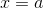, then the limit asapproachesexists, but the value of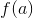does not.

For example, the functioncontains a removeable discontinuity at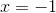. Notice that we could simplifyas follows: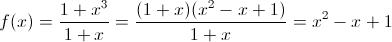, where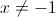.

Thus, we could say that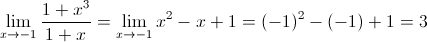.

As we can see, the limit ofexists at, even though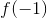is undefined.

What this means is thatwill look just like the parabola with the equation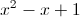EXCEPT when, where there will be a hole in the graph. However, if we were to just define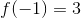, then we could essentially "remove" this discontinuity. Therefore, we can say that there is a removeable discontinuty at.

The functions, andhave discontinuities, but these discontinuities occur as vertical asymptotes, not holes, and thus are not considered removeable.

The functionsandare continuous over all the real values of; they have no discontinuities of any kind..